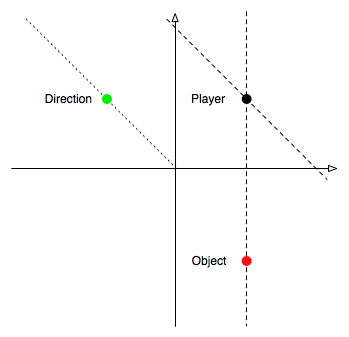• 您可以使用以下方法使用Math.atan2方法计算弧度角：public static double angleBetweenTwoPointsWithFixedPoint(double point1X,double point1Y,double point2X,double point2Y,double fixedX,double fixedY) {...

您可以使用以下方法使用Math.atan2方法计算弧度角：

public static double angleBetweenTwoPointsWithFixedPoint(double point1X,double point1Y,double point2X,double point2Y,double fixedX,double fixedY) {

double angle1 = Math.atan2(point1Y - fixedY,point1X - fixedX);

double angle2 = Math.atan2(point2Y - fixedY,point2X - fixedX);

return angle1 - angle2;

}

并用三点(使用Math.toDregrees将所得角度从弧度变换为度数)：

System.out.println(Math.toDegrees(

angleBetweenTwoPointsWithFixedPoint(0,// point 1's x and y

1,1,// point 2

1,0 // fixed point

)));

输出：90.0

随时可以在解决方案中使用Java的标准Point或Line2D类.这只是为了证明它的作品.

展开全文• 根据两个坐标点，计算两点之间角度 最常用的场景：地图上的标记点需要动态的改变角度 例子如下： 假如地图上有一条路线轨迹，你的标记点是一辆车的图标，你的车（标记点）在轨迹上运行的时候，如果不动态更改...

根据两个坐标点，计算出两点之间的角度

最常用的场景：地图上的标记点需要动态的改变角度

例子如下：

假如地图上有一条路线轨迹，你的标记点是一辆车的图标，你的车（标记点）在轨迹上运行的时候，如果不动态更改旋转角度的话，就会如下图所示，显然这用户体验是很差的。所以标记点移动的时候，就要算出两点之间的一个角度，然后动态的赋值给地图中标记点的旋转角度参数。

真正的效果应该是这样的废话不多说直接上代码

// 坐标1
let position1 = {
lng: 118.109673,
lat: 24.580435,

}
// 坐标2
let position2 = {
lng: 118.10961,
lat: 24.580648,
}
let valLng = position1.lng - position2.lng;
let valLat = position1.lat - position2.lat;

// 返回一个绝对值
let absoluteLng = Math.abs(valLng);
let absoluteLat = Math.abs(valLat);

// 取得三角形的斜边
let hypotenuse = Math.hypot(absoluteLng, absoluteLat);
// 计算弧度
let radina = Math.acos(absoluteLng / hypotenuse);

// 计算角度
let angle = Math.floor(radina * 180 / Math.PI);

console.log("两坐标点计算出的角度为：", angle)

两点之间的角度算出来了，其实还存在一个问题，就是y轴对称的点，他们计算出的角度是一样的，仔细看下面图中标识的点坐标点1与坐标点2-A的角度 = 坐标1与坐标点2-B的角度

小提示：

解决问题：那么就需要用到坐标系中的象限了，如下图注意看下面的两个例子，看是如何计算的

/**
* 例子1
* 旋转角度区间： 0~360* 标记点的方向： 由东向西
*/

// 计算出标记点真正旋转的角度
if (valLng > 0) {
if (valLat > 0) {
// 第三象限
angle = 360 - angle;
} else {
// 第二象限
angle = angle;
}
} else {
if (valLat > 0) {
//第四象限
angle = 180 + angle;
} else {
// 第一象限
angle = 180 - angle;
}
}
console.log("坐标点真正旋转的角度：", angle)

/**
* 例子2
* 旋转角度区间： 0~360* 标记点的方向： 由西向东
*/

// 计算出标记点真正旋转的角度
if (valLng > 0) {
if (valLat > 0) {
// 第三象限
angle = 180 - angle;
} else {
// 第二象限
angle = 180 + angle;
}
} else {
if (valLat > 0) {
//第四象限
angle = angle;
} else {
// 第一象限
angle = 360 - angle;
}
}
console.log("坐标点真正旋转的角度：", angle)

下面是整个计算的完整代码示例:

// 坐标1
let position1 = {
lng: 118.112193,
lat: 24.580612,

}
// 坐标2
let position2 = {
lng: 118.112494,
lat: 24.580883
}
let valLng = position1.lng - position2.lng;
let valLat = position1.lat - position2.lat;

// 返回一个绝对值
let absoluteLng = Math.abs(valLng);
let absoluteLat = Math.abs(valLat);

// 取得三角形的斜边
let hypotenuse = Math.hypot(absoluteLng, absoluteLat);
// 计算弧度
let radina = Math.acos(absoluteLng / hypotenuse);

// 计算角度
let angle = Math.floor(radina * 180 / Math.PI);

console.log("两坐标点计算出的角度为：", angle)

// 计算出标记点真正旋转的角度
if (valLng > 0) {
if (valLat > 0) {
// 第三象限
angle = 360 - angle;
} else {
// 第二象限
angle = angle;
}
} else {
if (valLat > 0) {
//第四象限
angle = 180 + angle;
} else {
// 第一象限
angle = 180 - angle;
}
}
console.log("坐标点真正旋转的角度：", angle)

好了，看不懂的我建议多看几次，仔细慢慢理解一下，其实你会发现很简单，有什么问题或者有更好的方法，欢迎留言指出 ~ / ~

展开全文js javascript
• I'm sorry if this question seems a really basic, but I cannot find a good answer online yet.I'm a little confused with vectors and how to use them in matlab. At the moment I have the following three p...I'm sorry if this question seems a really basic, but I cannot find a good answer online yet.

I'm a little confused with vectors and how to use them in matlab. At the moment I have the following three pair of coordinates (x and y): Person 1, The future location of Person 1 and Person 2. See: The three points in a 2d view

Now I want to calculate the angle between "the vector which goes from person 1 to person 2" and "the vector from person 1 to person 1 future". I've found some matlab functions which could do this with the vectors, but I am not sure I am actually using the right input for each vector.

So now my question is how can I use these coordinates (and the difference between them) to calculate the angle from the image?

解决方案

Although StefanM's solution is a pretty common solution to this, it is actually computationally expensive, but most importantly, incorrect when the vectors are tiny and/or the angle is near 0 or π -- it can actually result in angles that are slightly negative, or slightly exceed π.

Thus, it gives a false sense of robustness.

theta = acos(min(1,max(-1, a(:).' * b(:) / norm(a) / norm(b) )));

More robust, more correct, over 10× faster when run in a loop, and understandable by laymen without documentation, because it still agrees mostly with the "classical" formula.

展开全文• 我在游戏中有个向量 . 一个向量是玩家，一个向量是一个对象 .... 我想计算两条虚线之间角度 . 一条虚线连接玩家和物体，另一条线代表玩家从玩家所处的方向所朝向的方向 .目前，我正在这样做...

我在游戏中有两个向量 . 一个向量是玩家，一个向量是一个对象 . 我还有一个向量，指定玩家面对的方向 . 方向矢量没有z部分 . 这个点的大小为1，位于原点附近 .

我想计算士兵当前面对的方向和物体之间的角度，所以我可以正确地平移一些音频(仅限立体声) .

下图描述了我的问题 . 我想计算两条虚线之间的角度 . 一条虚线连接玩家和物体，另一条线代表玩家从玩家所处的方向所朝向的方向 .目前，我正在这样做(假设玩家，物体和方向都是3点，x，y和z的向量)：

Vector3d v1 = direction;

Vector3d v2 = object - player;

v1.normalise();

v2.normalise();

float angle = acos(dotProduct(v1, v2));

但它似乎给我不正确的结果 . 有什么建议？

代码测试：

Vector3d soldier = Vector3d(1.f, 1.f, 0.f);

Vector3d object = Vector3d(1.f, -1.f, 0.f);

Vector3d dir = Vector3d(1.f, 0.f, 0.f);

Vector3d v1 = dir;

Vector3d v2 = object - soldier;

long steps = 360;

for (long step = 0; step < steps; step++) {

float rad = (float)step * (M_PI / 180.f);

v1.normalise();

float dx = dotProduct(v2, v1);

float dy = dotProduct(v2, soldier);

float vangle = atan2(dx, dy);

}

展开全文• 坡度和角度计算公式？坡度的表示方法有百分比法、度数法、密位法和分数法四种，其中以百分比法和度数法较为常用.(1) 百分比法 表示坡度最为常用的方法，即两点.要详细点的 1度等于多少秒多少分 50度41分20秒等于多少...
• 最低0.27元/天开通百度文库会员，可在文库查看完整内容> 原发布者：design_ycl ?个向量的夹角的定义：对于非零向量，，作称为向量，的夹角，当=0时，，同向...给的是坐标，要求步骤详细，多谢夹角为α=arccos...两个向量的夹角公式
• 反余弦计算方式：private static final double EARTH_RADIUS = 6371000; // 平均半径,单位：m；不是赤道半径。赤道为6378左右public static double getDistance(Double lat1,Double lng1,Double lat2,Double lng2) {...
• 问题描述： 平常为了得出地理位置上两点的实际...在此之前，需要计算出球面上两条直线夹角以及两点在球面上的距离。 1）球面上两条直线夹角 方法一：简易版，将球面弧线看成是直角坐标系下的直线，采用向量乘积python 算法
• 方法一：/***计算地球上任意两点(经纬度)距离**@paramlong1*第一点经度*@paramlat1*第一点纬度*@paramlong2*第二点经度*@paramlat2*第二点纬度*@return返回距离单位：米*/publicstaticdoubleDistan...
• 两点距离(1.4142135623730951)select st_distance(point(0,0),point(1,1));select st_distance(point (120.10591, 30.30163),point(120.13026,30.25961));mysql 5.6 添加#2.两点球面距离(157249.0357231545m)select ...
• 最近做项目需要计算用户和商家之间的距离，并要求在Listview中显示具体距离。想了一下大致思路，首先是拿到当前用户的经纬度...一种是计算两点之间的球面距离；两种计算方式我做了一下比较，当两点的距离较近时使用...
• 1、根据个gps的信息计算距离和角度 2、根据一个gps和距离、角度进而求终点的位置 博客地址： 添加链接描述 添加链接描述 添加链接描述 C++代码： 头文件： #ifndef MYBIGCIRCLECLASS_H #define MYBIGCIRCLECLASS...c++
• PHP根据两点间的经纬度计算距离，php两点经纬度计算这是一个不错的示例，直接贴代码，首先要知道纬度值、经度值/*** @desc 根据两点间的经纬度计算距离* @param float $lat 纬度值* @param float$lng 经度值*/...
• 概述：本道作业题是沃捍瞻同学的课后练习，分享的知识是圆的角度公式，指导老师为晏老师，涉及到的知识涵盖：【圆角度计算公式是什么】-圆的角度公式-数学，下面是沃捍瞻作业题的详细。题目：【圆角度计算...
• Math.PI 表示一个圆的周长与直径的比例，约为 3.141592653589793，其实就是我们所说的圆周率 π Math.sin(x) //x 的正玄值。...弧度的计算公式为： 2PI/360角度； 弧度 = 角度 ×（PI/180）； 30° 角度 的弧度 = 3html5 javascript css
• 一直等腰三角形其中一个角的度数为30度 组成这个角的条边唱分别是1.5米 .1、计算 excel中，对于角度的运算是用“弧度”的，所以计算时要注意，若是“角度”，则要进行转换，入sin30°，公式应为=sin(30/180...
• 根据两点经纬度计算距离

千次阅读 2021-01-14 04:50:44
如果以0度经线为基 准，那么根据地球表面任意两点的经纬度就可以计算出这两点间的地表距离（这里忽略地球表面地形对计算带来的误差，仅仅是理论上的估算值）。设第一点A的经 纬度为(LonA, LatA)，第二点B的经纬度为...
• 计算两平面的夹角面面角向量的积代码部分 面面角 平面的夹角为所成角中较小的角。 n1为plane1的法向量，如plane1的平面方程为a1x + b1y + c1z + d1 = 0，n1的向量表达式为(a1, b1, c1)。 向量的积 空间中有...
• 计算3个地理坐标点之间夹角背景计算3个地理坐标点之间夹角 背景  来了个需求，需要知道大疆OSDK执行WaypointV2Mission时候，航点之间的转向角是顺时针还是逆时针的(一开始以为WaypointV2是随机顺逆旋转到下一个...c++
• 已知地球上任意两点（lng1，lat1），（lng2， lat2）的经纬度坐标，求两点间的距离可以利用 haversine 公式： 首先先将经纬度坐标的角度化成弧度（rlng1，rlat1），（rlng2，rlat2) 利用如下公式： 其中 a ...python haversine geopy 两点经纬度计算距离
• 《材料力学的基本计算公式-材料力学弯曲公式》由会员分享，可在线阅读，更多相关《材料力学的基本计算公式-材料力学弯曲公式(9页珍藏版)》请在人人文库网上搜索。1、材料力学的基本计算公式外力偶矩计算公式 (P功率...
• 本文给出了求解球面上两点球面距离的原理与C++实现代码，可以计算球面两点的欧式距离（弦长）、圆心角、球面距离。c++
• 坡度计算公式图解

万次阅读 2020-12-23 03:49:57
管道坡度计算公式管道坡度计算公式特 征码[ 标签: 特征码 ]如何计算坡度计算公式？我国对排水坡度计算公式怎么样？基 本概况如何？以下是坡度比基本内容如下：我们通过本网站建筑知识专栏的知识整理， 梳理坡度计算...
• matlab求两直线的夹角

千次阅读 2020-12-21 01:31:30
基于matlab求解初等几何问题lyqmath0引言在工程应用、科学计算等领域，计算两条直线的交点是一个较为基础的问题。一般的应该考虑到直线方程的写法和求解交点的方式。这里给出一个具体的处理方式，其基本思想是：首先...
• 毛利率有多种分类方式，按商品大类分，有单项商品毛利率、大类商品毛利率、综合商品...中文名毛利率计算公式阐述不含进项税额的单价计算确定的类别相关词汇决定因素市场竞争等毛利率计算公式分类方式编辑语音计算...
• 做建模或者研究空间数据，可能会遇到“根据经纬度计算两点间的球面距离”的问题，网上的资料很多，都是各种公式推导，但是一旦按公式编程计算，很可能得不到正确的距离。根本原因是在“角度-弧度的转化”与“软件...
• 如题，已经知道圆心和两点，画出两点间的圆弧，思路，先分别求出原点到两点向量和x轴夹角余弦值，然后求反余弦得出角度，这里要注意的是，求出的向量的夹角是按几何坐标系中定义的逆时针，然后用DrawArc()函数画...
• 弧长计算公式

千次阅读 2020-12-20 11:37:44
弧长的计算公式 弧长公式:n 是圆心角度数，r 是半径，a 是圆心角弧度。公式 l = n(圆心角)x π(圆周率)x r(半径)/180 在半径是 R 的圆中，因为 360°的圆心角所对的弧长就等于圆周长 C=2πR，所以 n°圆心角所对的...圆弧周长公式...